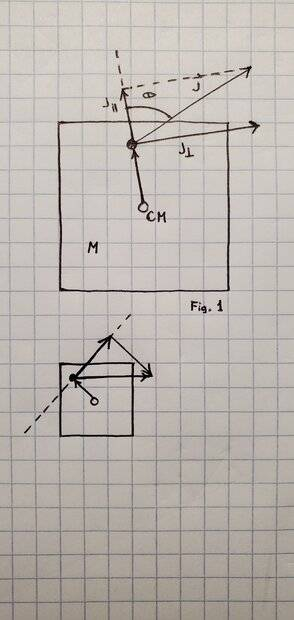# Solving for the trajectory of the center of mass

• I
• nDever

#### nDever

I'm working on the physics engine component of a game engine I'm building, and I need some guidance with this particular situation.

Consider a square with mass M that is free to translate in the xy plane and free to rotate about any axis perpendicular to the page (Fig. 1)

If a linear impulse J is applied at a point above the center of mass (CM) as shown below, I know there must be some angular impulse (momentary torque) generated since there is a component of J that is perpendicular to the displacement vector from CM. I imagine this angular impulse will tend to rotate the square clockwise.

However, I can also imagine that the CM will also undergo translation since the square is not constrained. How would I go about computing the overall rotational + translational motion of this system?•Delta2
The linear motion is the easiest since overall momentum conservation gives
$$\frac{d\vec p}{dt} = m\dot{\vec v}_{com} = \vec F_{tot}$$
The rotational part can be slightly trickier due to the overall acceleration of the system. You should be able to do it by overall angular momentum, preferably relative to the CoM in the comoving frame.

•vanhees71